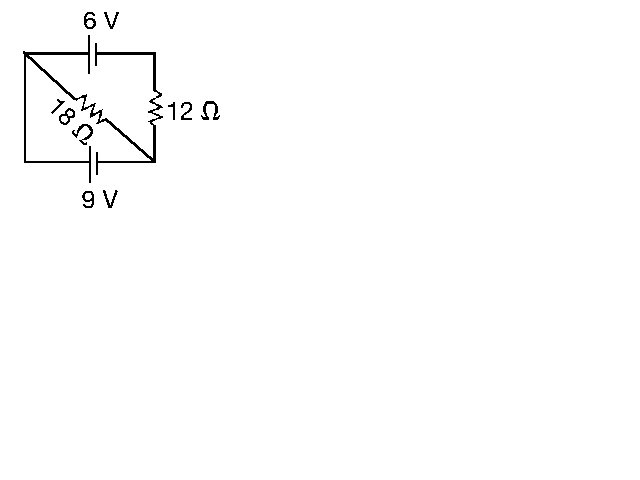Practice Exam 2, Ch. 18, 19

NOTE: ê means ohm. æ means micro.

Page 1

1. A total of 2.0X1013 protons pass a given point in 15 s. What is the current?

a. 1.3 mA

b. 1.3 A

c. 0.21 æA

d. 3.2 æA

2. What is the resistance of 1.0 m of no. 18 copper wire (diameter 0.40 in)? (The resistivity of copper is 1.69X10-8 êúm.)

a. 0.00012 ê

b. 0.00021 ê

c. 0.0012 ê

d. 0.0021 ê

3. What is 1 ê equivalent to?

a. 1 J/s

b. 1 W/A

c. 1 VúA

d. 1 V/A

4. Consider two copper wires. One has twice the length and twice the cross-sectional area of the other. How do the resistances of these two wires compare?

a. Both wires have the same resistance.

b. The longer wire has twice the resistance of the shorter wire.

c. The longer wire has four times the resistance of the shorter wire.

d. None of the above.

5. Consider two copper wires. One has twice the length of the other. How do the resistivities of these two wires compare?

a. Both wires have the same resistivity.

b. The longer wire has twice the resistivity of the shorter wire.

c. The longer wire has four times the resistivity of the shorter wire.

d. None of the above.

6. What is the resistance of a circular rod 1.0 cm in diameter and 45 m long, if the resistivity is 1.4X10-8 êúm?

a. 0.0063 ê

b. 0.0080 ê

c. 0.80 ê

d. 6.3 ê

7. A 200-ê resistor is rated at 1/4 W. What is the maximum voltage?

a. 0.71 V

b. 7.1 V

c. 50 V

d. 0.25 V

Page 2

8. If the voltage across a circuit of constant resistance is doubled, the power dissipated by that circuit will

b. double.

c. decrease to one half.

d. decrease to one fourth.

9. 4.00 A is flowing through an 8.00 ê resistor. How much power is being dissipated?

a. 32.0 W

b. 128 W

c. 256 W

d. 1.02 kW

10. How much energy does a 100 W light bulb use in 8.0 hours?

a. 0.0080 kWh

b. 0.80 kWh

c. 13 kWh

d. 800 kWh

11. Three identical resistors are connected in series to a battery. If the current of 12 A flows from the battery, how much current flows through any one of the resistors?

a. 12 A

b. 4 A

c. 36 A

d. zero

12. When two or more resistors are connected in parallel to a battery,

a. the voltage across each resistor is the same.

b. the total current flowing from the battery equals the sum of the currents flowing through each resistor.

c. the equivalent resistance of the combination is less than the resistance of any one of the resistors.

d. all of the above.

13. As more resistors are added in parallel to a constant voltage source, the power supplied by the source

a. increases.

b. decreases.

c. does not change.

d. increases for a time and then starts to decrease.

14. A combination of 2.0 ê in series with 4.0 ê is connected in parallel with 3.0 ê. What is the equivalent resistance?

a. 2.0 ê

b. 3.0 ê

c. 4.0 ê

d. 9.0 ê

Page 3

15. Kirchhoff's voltage rule for a closed loop is an example of

a. conservation of energy.

b. conservation of charge.

c. conservation of momentum.

d. none of the above.

16. What current flows in the 18 ê resistor?

a. 0.25 A

b. 0.50 A

c. 0.75 A

d. 1.0 A17. A 22 A current flows into a parallel combination of a 4.0 ê, 6.0 ê, and 12 ê resistors. What current flows through the 6.0 ê resistor?

a. 18 A

b. 7.3 A

c. 3.7 A

d. None of the above.

Page 4

18. When two or more capacitors are connected in series to a battery,

a. the total voltage across the combination is the algebraic sum of the voltages across the individual capacitors.

b. each capacitor carries the same amount of charge.

c. the equivalent capacitance of the combination is less than the capacitance of any of the capacitors.

d. All of the above.

19. A 1.0-æF and a 2.0-æF capacitor are connected in series across a 3.0-V battery. What is the voltage across the 2.0-æF capacitor?

a. 3.0 V

b. 2.0 V

c. 1.0 V

d. zero

20. A 2.0-æF capacitor is charged though a 50,000-ê resistor. How long does it take for the capacitor to reach 90% of full charge?

a. 0.90 s

b. 0.23 s

c. 2.2 s

d. 2.3 s

21. A resistor and a capacitor are connected in series to an ideal battery of constant terminal voltage. When this system reaches its steady-state, the voltage across the resistor is

a. greater than the battery's terminal voltage.

b. less than the battery's terminal voltage, but greater than zero.

c. equal to the battery's terminal voltage.

d. zero.

Page 1

1. c

2. b

3. d

4. a

5. a

6. b

7. b

8. a

9. b

10. b

11. a

12. d

13. a

14. a

15. a

16. b

17. b

18. d

19. c

20. b

21. d